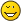# Tetration Forum

Full Version: Expansion of base-e pentation
You're currently viewing a stripped down version of our content. View the full version with proper formatting.
Pages: 1 2
So I have been revamping the functions I use for tetration, and I recently solved all the mumbo-jumbo to use tetration as the function in the NaturalIterate function. So now you can do:

Code:
```<<Tetration` NaturalIterate[Series[Tetrate[E, x], {x, 0, 3}], z]```

I'm still working on doing this for other bases, but this is enough to get an expansion of pentation about zero.

What follows below is InverseSeries[...] of the output from above. In other words, the above gives the coefficients of the base-e penta-logarithm about (0), and the following gives the coefficients of the base-e penta-exponential about (-1).
Code:
```0, 0.997386001614238200000, -0.044854069033065140000, 0.008127184531878105000, 0.045268576293608810000, -0.009169795166599723000, 0.000529626080101428000, 0.003682350459440369500, -0.001300714479652927000, 0.000136554270543782140, 0.000349632018705509600, -0.000212903018660854500, 0.000030850789704285015, 0.000053653522961255240, -0.000028243223065159680, -0.000003800898968414997, 0.000000972449120890964, 0.000005775482651540000, 0.000010790317715530437, -0.000029357772002764790, 0.000020775705975594905```

So, what I'm wondering is, how do I turn this into an expansion about 3i?
Oh wow you did Intuitive iteration on the intuitive iteration of exp?
(10/24/2009, 10:13 AM)bo198214 Wrote: [ -> ]Oh wow you did Intuitive iteration on the intuitive iteration of exp?

Yes, It was quite a nightmare.I downloaded and open "tetration-0.8.zip" but I this compression doesn't have any file after folder "tetration"!??
Sorry, try again.

PS. You might have to rewrite the 'PackageFile' function.
I don't get run for errors (invalid characters) using matlab r2009a. but this matlab doesn't have "PackageFile" function.
do you fix? if not then I ask if you convert this file from matlab to mathematica 7?
please.(10/25/2009, 12:14 PM)nuninho1980 Wrote: [ -> ]I don't get run for errors (invalid characters) using matlab r2009a. but this matlab doesn't have "PackageFile" function.
do you fix? if not then I ask if you convert this file from matlab to mathematica 7?
please.This is a Mathematica package, it will not work in matlab...

I'm not talking about a system function, I'm talking about one of my functions. In the file Tetration/Tetration.m there is a function called 'PackageFile' that imports files based on an absolute path. Depending on where you install it, you will probably have to change this to suit your installation.
thanks! but the height of tetration is integer only?
(10/29/2009, 10:18 PM)nuninho1980 Wrote: [ -> ]thanks! but the height of tetration is integer only?
Nope, it works for complex values, but only for base E. I'm still working on making it easier to use other bases.
(10/24/2009, 04:30 AM)andydude Wrote: [ -> ].....
I'm still working on doing this for other bases, but this is enough to get an expansion of pentation about zero.

What follows below is InverseSeries[...] of the output from above. In other words, the above gives the coefficients of the base-e penta-logarithm about (0), and the following gives the coefficients of the base-e penta-exponential about (-1).

[attachment=790]

Code:
```\r kneser.gp \r pentation.gp gp > init;loop;   /* 13 sexp(z) loops .... */ gp > genpent /* generate pentation base e */ complex sexp Taylor series centered at -1.8503545290271814184834459502910 pentation base        2.71828182845904523536029 pentation(-0.5)       0.491054338635648197413514 sexp fixed point      -1.85035452902718141848345 sexp slope at fixed   6.46067129568183939020883 pentation period      3.36767615671259898023746*I pentation singularity -2.31527062760141112146561 + 1.68383807835629949011873*I pentation precision, via sexp(pent(-0.5))-pent(0.5)                       -9.69394178147793704836998 E-21 gp > ploth(t=-10,1.5,pent(t));```

So what's all this telling you? Its telling you that the fixed point for sexp(z) to generate pentation base e, pfixed, is -1.85035.... Its telling you the slope of sexp(pfixed)=-6.46067... which leads to the pentation complex periodic period, pperiod=3.367*I. At imag(z)=0, the function is real valued for all z. Here is the graph, generated by that last line ploth line. Notice, it starts out at the real valued fixed point, pfixed, and then grows somewhat intermittantly, with pent(-inf)=-1.85..., pent(-2)=-1, pent(-1)=0, and pent(0)=1, and pent(2)=e, and pent(3)=sexp(e).
[attachment=791]

At imag(z)=imag(pperiod/2), there is another real valued line, starting at the fixed point and growing towards -infinity, which is the first singularity that occurs at -2.315 + 1.6838*I. Then there is a slew of singularities after that, corresponding to sexp(z)=-2,-3,-4,-5 ....
[attachment=792]

Continuing, to generate the Taylor series, centered at pentation(z=-1). Notice that the first term of the Taylor series is almost zero, but not quite due to precision errors. The pentation taylor series is accurate to approximately 20 decimal digits. More accurate results are possible by setting gp's precision to "\p 134". Then type in the sexp initialization command, "init;loop". Later, I will post pentation plots for other bases. So far, I've only had time to try bases between B=1.6 and B=e, all of which worked fine. I'll post more later. Here are the Taylor series terms, followed by Andy's terms for historical comparison.
- Sheldon
Code:
```gp > pentaylor(-1,1) gp > for (s=1,30,print(real(ptseries[s]))) 6.7242536178550628294755514087 E-24 0.99727185142263340743455208346122 -0.045007215859218115832617467992327 0.0088901369292365764437286761921372 0.045713734782598722205971510001068 -0.010706554884752458976051797391420 0.00011329335331439235574805971805731 0.0051620130076806122858704585184006 -0.0012422756898373028878826856222621 -0.00067376885079665208568672130450693 0.00050296665968765950574361816768155 0.000039905534193068199638492988158461 -0.000094623078715532686231662582532929 0.000026746817775170179559855402990613 0.000015560915176630839373361742908680 -0.000014806164180600879049897255662325 -0.0000010862859329576915398646271649914 0.0000059140073162222162194013397871868 -0.00000071091367653831526613315587588080 -0.0000017666311876111783264226773258896 0.00000051445590441872869647430881209296 0.00000036270009896115685098739030842790 -0.00000020971694575358607315821487792583 -0.000000021225391058732913781072384245741 0.000000064546351710396107513893801263449 -0.000000022236468044317568271338433775775 -0.000000015692632671055194971436603480275 0.000000013400661392837577306043949458764 0.0000000026987924084642996469868738785694 -0.0000000049614802252464409094532195966523```

Andy's results are pretty reasonable, all considering, but only for the first few terms. Here are Andy's coefficients for historical record.
Code:
```0, 0.997386001614238200000, -0.044854069033065140000, 0.008127184531878105000, 0.045268576293608810000, -0.009169795166599723000, 0.000529626080101428000, 0.003682350459440369500, -0.001300714479652927000, 0.000136554270543782140, 0.000349632018705509600, -0.000212903018660854500, 0.000030850789704285015, 0.000053653522961255240, -0.000028243223065159680, -0.000003800898968414997, 0.000000972449120890964 0.000005775482651540000, 0.000010790317715530437, -0.000029357772002764790, 0.000020775705975594905```
Pages: 1 2